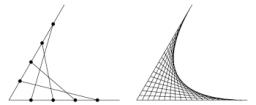# Summands 4213

Divide the number 28 into two summands so that their product is maximal.

a =  14
b =  14

### Step-by-step explanation:Did you find an error or inaccuracy? Feel free to write us. Thank you!

Tips for related online calculators
Do you have a linear equation or system of equations and looking for its solution? Or do you have a quadratic equation?